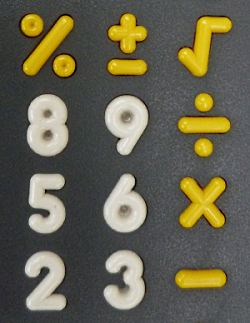calculator.org

# Precedence

### What precedence does your calculator use?Precedence refers to the order in which arithmetic operations are performed. It is easy to check whether a calculator uses algebraic precedence, for example if multiplication is done before addition. Caculate the following:

2 + 3 X 4

If the answer is 20 the calculator is simply applying each arithmetic operator as it is entered, so the expression is interpreted as (2 + 3) X 4. Very simple four function calculators tend to behave this way. If the answer is 14 the calculator is using algebraic precedence. The answer is different because the multiplication operation was completed before the addition, according to the expression 2 + (3 X 4). Scientific calculators always use algebraic precedence, which means that binary operators are always applied in the order: exponents and roots, then multiplication and division, and finally addition and subtraction. Of course any brackets trump all other operators.

In order to make this work, the calculator has to store the addition operator and keep it pending while it waits to see if you supply a multiplication or other higher priority operator. Obviously the logic required to achieve this is not trivial, which is why cheaper or less sophisticated arithmetic calculators often don't have algebraic precedence. It is easier to just apply each operator as it is input.

At school we may be taught a mnemonic to remember the correct order of arithmetic operators for hand calculations (a discipline that is being practiced less and less as a result of increasing reliance on pocket calculators). One of these is BODMAS, standing for Brackets, Orders, Division, Multiplication, Addition, Subtraction. There are variations of this, all resulting in words that are equally nonsensical, because of the equal precedence of division and multiplication, and of addition and subtraction. A more useful mnemonic, in the sense that it does actually aid the memory, is "My Dear Aunt Sally" or "Please Excuse My Dear Aunt Sally", where the first letters of each word stand for Parentheses, Exponentiation, Multiplication, Division, Addition and Subtraction.

If you are lucky enough to be using a Reverse Polish Notation (RPN) calculator then precedence is not an issue, because in fact you are implicitly deciding the precedence as you enter the values. If you want to try this with our Calc software, remember that you can switch the algebraic precedence on or off in the General menu.